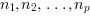Scilab Home page | Wiki | Bug tracker | Forge | Mailing list archives | ATOMS | File exchange
Please login or create an account
Scilab 5.4.0
Change language to: English - Português - 日本語 - Русский

Please note that the recommended version of Scilab is 6.1.0. This page might be outdated.
See the recommended documentation of this function

# dct

Discrete cosine transform.

# idct

Inverse discrete cosine transform.

### Calling Sequence

```X=dct(A [,sign] [,option])
X=dct(A,sign,selection [,option])
X=dct(A,sign,dims,incr [,option])
X=idct(A [,option])
X=idct(A,selection [,option])
X=idct(A,dims,incr [,option])```

### Arguments

A

a real or complex vector or real or complex array (vector, matrix or N-D array.

X
a real or complex array with same shape as `A`.
sign
an integer. with possible values `1` or `-1`. Select direct or inverse transform. The default value is `-1` (direct transform).
selection
a vector containing index on `A` array dimensions. See the Description part for details.
dims
a vector of positive numbers with integer values, or a vector of positive integers. See the Description part for details.

Each element must be a divisor of the total number of elements of `A`.

The product of the elements must be less than the total number of elements of `A`.

incr
a vector of positive numbers with integer values, or a vector of positive integers. See the Description part for details.

`incr` must have the same number of elements than `dims`.

Each element must be a divisor of the total number of elements of `A`.

The `incr` elements must be in strictly increasing order.

option
a character string. with possible values `"dct1"`, `"dct2"`, `"dct4"` or `"dct"` for direct transform and `"dct1"`, `"dct3"`, `"dct4"` or `"idct"` for inverse transform. The default value is `"dct"` for direct transform and `"idct"` for inverse transform. See the Description part for details.

### Transform description

This function realizes direct or inverse 1-D or N-D Discrete Cosine Transforms with shift depending on the `option` parameter value. For a 1-D arrayof length:

• For `"dct1"` the function computes the unnormalized DCT-I transform:• For `"dct2"` the function computes the unnormalized DCT-II transform:• For `"dct3"` the function computes the unnormalized DCT-III transform:• For `"dct4"` the function computes the unnormalized DCT-IV transform:• For `"dct"` the function computes the normalized DCT-II transform:• For `"idct"` the function computes the normalized DCT-III transform:The multi-dimensional DCT transforms , in general, are the separable product of the given 1d transform along each dimension of the array. For unnormalized transforms , computing the forward followed by the backward/inverse multi-dimensional transform will result in the original array scaled by the product of the dimension sizes.

The normalized multi-dimensional DCT transform of an array `A` with dimensionsis given byThe normalized multi-dimensional DCT inverse transform of an array `A` with dimensionsis given by### Syntax description

Short syntax
direct

`X=dct(A,-1 [,option])` or `X=dct(A [,option])` gives a direct transform according to the option value. The default is normalized DCT-II direct transform.

If `A` is a vector (only one dimension greater than 1) a 1-d transform is performed and in the other cases a n-dimensional transform is done.

(the `-1` argument refers to the sign of the exponent..., NOT to "inverse"),

inverse

`X=dct(A,1 [,option])` or `X=idct(A [,option])`performs the inverse transform.

If `A` is a vector (only one dimension greater than 1) a 1-d transform is performed and in the other cases a n-dimensional transform is done.

Long syntax for DCT along specified dimensions
• `X=dct(A,sign,selection [,option])` allows to perform efficiently all direct or inverse dct of the "slices" of `A` along selected dimensions.

For example, if `A` is a 3-D array `X=dct(A,-1,2)` is equivalent to:

```for i1=1:size(A,1),
for i3=1:size(A,3),
X(i1,:,i3)=dct(A(i1,:,i3),-1);
end
end```

and `X=dct(A,-1,[1 3])` is equivalent to:

```for i2=1:size(A,2),
X(:,i2,:)=dct(A(:,i2,:),-1);
end```
• `X=dct(A,sign,dims,incr)` is an old syntax that also allows to perform all direct or inverse dct of the slices of `A` along selected dimensions.

For example, if `A` is an array with `n1*n2*n3` elements `X=dct(A,-1,n1,1)` is equivalent to `X=dct(matrix(A,[n1,n2,n3]),-1,1)`. and `X=dct(A,-1,[n1 n3],[1 n1*n2])` is equivalent to `X=dct(matrix(A,[n1,n2,n3]),-1,[1,3])`.

### Optimizing dct

Remark: function automatically stores his last parameters in memory to re-use it in a second time. This improves greatly the time computation when consecutives calls (with same parameters) are performed.

It is possible to go further in dct optimization using get_fftw_wisdom, set_fftw_wisdom functions.

### Algorithms

This function uses the fftw3 library.

### Examples

1-D dct

```//Frequency components of a signal
//----------------------------------
// build a sampled at 1000hz  containing pure frequencies
// at 50 and 70 Hz
sample_rate=1000;
t = 0:1/sample_rate:0.6;
N=size(t,'*'); //number of samples
s=sin(2*%pi*50*t)+sin(2*%pi*70*t+%pi/4)+grand(1,N,'nor',0,1);
d=dct(s);
// zero low energy components
d(abs(d)<1)=0;
size(find(y1<>0),'*') //only 30 non zero coefficients out of 600
clf;plot(s,'b'),plot(dct(d,1),'r')```

2-D dct

```----------------------------------
x=-2:0.1:2;
A=eval3d(milk_drop,x,x);
d=dct(A);
d(abs(d)<1)=0;
size(find(d1<>0),'*')
A1=dct(d,1);
clf();fig=gcf();fig.color_map=graycolormap(128);
subplot(121),grayplot(x,x,A)
subplot(122),grayplot(x,x,A1)```

### See Also

• fft — Transformée de Fourier discrète rapide.
• dst — Discrete sine transform.
• fftw_flags — choix de la méthode pour la sélection de l'algorithme de planification pour la fft
• get_fftw_wisdom — retourne le wisdom fftw
• set_fftw_wisdom — charge un wisdom fftw
• fftw_forget_wisdom — Re-initialise le wisdom fftw

### Bibliography

Matteo Frigo and Steven G. Johnson, "FFTW Documentation" http://www.fftw.org/#documentation

### Comments

Add a comment:
Please login to comment this page.

 Report an issue << fft transforms dft >>

 Scilab EnterprisesCopyright (c) 2011-2017 (Scilab Enterprises)Copyright (c) 1989-2012 (INRIA)Copyright (c) 1989-2007 (ENPC)with contributors Last updated:Mon Oct 01 17:37:18 CEST 2012